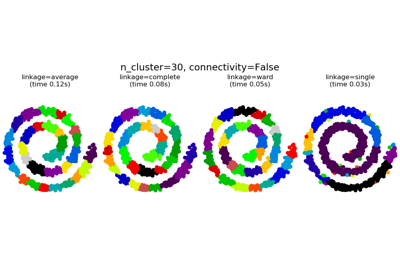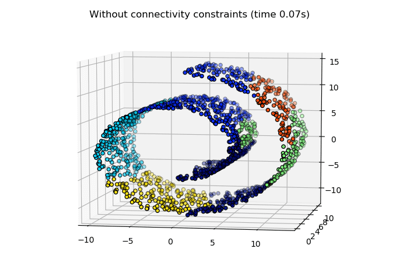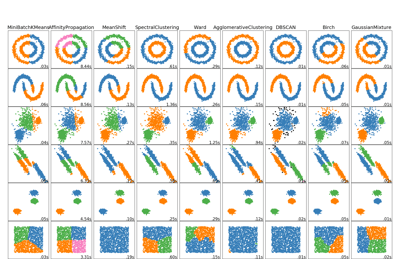/scikit-learn

# sklearn.neighbors.kneighbors_graph

`sklearn.neighbors.kneighbors_graph(X, n_neighbors, mode=’connectivity’, metric=’minkowski’, p=2, metric_params=None, include_self=False, n_jobs=None)` [source]

Computes the (weighted) graph of k-Neighbors for points in X

Read more in the User Guide.

Parameters: `X : array-like or BallTree, shape = [n_samples, n_features]` Sample data, in the form of a numpy array or a precomputed `BallTree`. `n_neighbors : int` Number of neighbors for each sample. `mode : {‘connectivity’, ‘distance’}, optional` Type of returned matrix: ‘connectivity’ will return the connectivity matrix with ones and zeros, and ‘distance’ will return the distances between neighbors according to the given metric. `metric : string, default ‘minkowski’` The distance metric used to calculate the k-Neighbors for each sample point. The DistanceMetric class gives a list of available metrics. The default distance is ‘euclidean’ (‘minkowski’ metric with the p param equal to 2.) `p : int, default 2` Power parameter for the Minkowski metric. When p = 1, this is equivalent to using manhattan_distance (l1), and euclidean_distance (l2) for p = 2. For arbitrary p, minkowski_distance (l_p) is used. `metric_params : dict, optional` additional keyword arguments for the metric function. `include_self : bool, default=False.` Whether or not to mark each sample as the first nearest neighbor to itself. If `None`, then True is used for mode=’connectivity’ and False for mode=’distance’ as this will preserve backwards compatibility. `n_jobs : int or None, optional (default=None)` The number of parallel jobs to run for neighbors search. `None` means 1 unless in a `joblib.parallel_backend` context. `-1` means using all processors. See Glossary for more details. `A : sparse matrix in CSR format, shape = [n_samples, n_samples]` A[i, j] is assigned the weight of edge that connects i to j.

#### Examples

```>>> X = [, , ]
>>> from sklearn.neighbors import kneighbors_graph
>>> A = kneighbors_graph(X, 2, mode='connectivity', include_self=True)
>>> A.toarray()
array([[1., 0., 1.],
[0., 1., 1.],
[1., 0., 1.]])
```

## Examples using `sklearn.neighbors.kneighbors_graph`Agglomerative clustering with and without structureHierarchical clustering: structured vs unstructured wardComparing different clustering algorithms on toy datasets